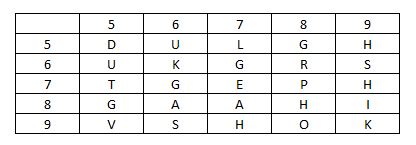# SSC CHSL 3 Feb 2017 Afternoon Shift

Instructions

For the following questions answer them individually

Question 91

In a certain code language, "TOMATO" is written as "023402" and "ORIONL" is written as "275269". How is "NORMAL" written in that code language?

Question 92

Find the Missing Number from the given Table

Question 93

If ‘+' means ‘minus', ‘x' means ‘divided by', ‘÷' means ‘plus' and ‘-' means‘multiplied by', then
104 × 13 + 9 - 5 ÷ 6 = ?

Question 94

In the following question, which one set of letters when sequentially placed at the gaps in the given letter series shall complete it?
a_c_a_cb_ccb

Question 95

Arjun is facing south, then he turns 45° right and goes 50 m, then turns north-west to move 50 m and from there 50 m to west. In which direction/place is he from his original place?

Question 96

A word is represented by only one set of numbers as given in any one of the alternatives. The sets of numbers given in the alternatives are represented by two classes of alphabets as shown in the given two matrices. The columns and rows of Matrix-I are numbered from 0 to 4 and that of Matrix-II are numbered from 5 to 9. A letter from these matrices can be represented first by its row and next by its column, for example, 'R' can be represented by 02, 20 etc. and `U' can be represented by 56, 65 etc. Similarly, you have to identify the set for the word 'ROUGH'.

MATRIX 1

MATRIX 2Question 97

Introducing a boy, a girl said, "He is the son of the sister of my mother's brother". How is that boy related to that girl?

Question 98

If a mirror is placed on the line MN, then which of the answer figures is the right image of the given figure?

Question 99

Identify the diagram that best represents the relationship among the given classes.
Metal, Oxygen, Copper

Question 100

A piece of paper is folded and punched as shown below in the question figures. From the given answer figures, indicate how it will appear when opened.

OR